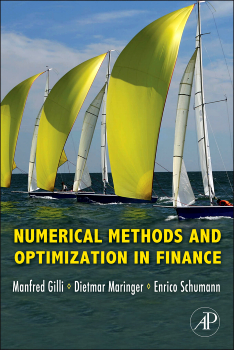# Review of “Numerical Methods and Optimization in Finance” by Gilli, Maringer and Schumann## Previously

This book and the associated R package were introduced before.

## Executive Summary

A very nice — and enlightening — discussion of a wide range of topics.

## Principles

The Introduction to the book sets out 5 principles.  This is probably the most important part of the book.  The principles are:

1. We don’t know much in finance.
2. Don’t be scared.
3. Judge the quality of a solution with respect to the application.
4. Don’t translate math literally into computer code.
5. Go experiment.

The “we” in Principle 1 is not a personal we, but the collective we:

… there is a vast body of theory.  But when we look at the empirical track record of much of this theory — let us say there remains room for improvement.

Another quote on this principle is:

Some textbooks in finance give the impression that, for example, “if you want to do portfolio optimization, this is what you need to do.”  No, please.  It should be “This is one possibility, and if you have a better idea, please try.”  This is all the more true since many models in finance were not motivated by empirical usefulness, but rather mathematical elegance or mere tractability.

A blog post that relates to ignorance is “The shadow and light of models”.

Principle 2 is about being intimidated by highly formal and complex mathematics in finance.  Some simple computer code can be an antidote for the intimidation.

The final principal is explained by:

When you implement an idea, try things, experiment, work with your data, go wild.  The tools described in this book will, we hope, help to better test and implement ideas.  Eventually, it is the applications that matter; not the tools.  We do not need a perfect hammer, the question is where to put the nail.

## Computing

The book uses both R and Matlab.  It is a bit of a Rosetta stone for those who know one language and are interested in the other.

## Modeling

In sum, we can roughly divide our modeling into two steps: from reality to the model, and then from the model to its numerical solution.  Unfortunately, large parts of the computational finance literature seem only concerned with assessing the quality of the second step …

An exception is Emanuel Derman’s book Models. Behaving. Badly.

## Outline

The book has three parts:

• Fundamentals
• Simulation
• Optimization

### Fundamentals

The topics in this section include:

• numerical analysis
• matrix operations for least squares and such
• differential equations and options models
• binomial trees
• numerical integration

The last of these, while logically fundamental, is given a deeper treatment in the book and is actually the last topic of the optimization part.

### Simulation

The topics of this section include:

• pseudo-random number generation
• quasi-Monte Carlo
• Markov chain Monte Carlo
• garch simulation
• agent-based models

### Optimization

This part starts with a discussion of classical optimization — derivative-based methods and the Nelder-Mead simplex algorithm.

Then it moves to heuristic optimization, which is the focus of this part.  It makes a distinction between two types of methods:

• trajectory
• population-based

Trajectory means that there is a single active solution and a search from there for a better solution.  Simulated annealing is the most widely known in this class.

Genetic algorithms are the best known of the population-based methods.  Particle swarm optimization is another example.

There is a chapter specifically on portfolio optimization.  The book hits upon a few of the tricks that make the Portfolio Probe optimization engine fast.

Other applications of optimization that are discussed include:

• the Nelson-Siegel model
• robust regression

## Getting it

To install the R package on your machine, then (assuming you have a connection to the internet) do:

`install.packages("NMOF")`
This entry was posted in Book review, Quant finance, R language and tagged , . Bookmark the permalink.